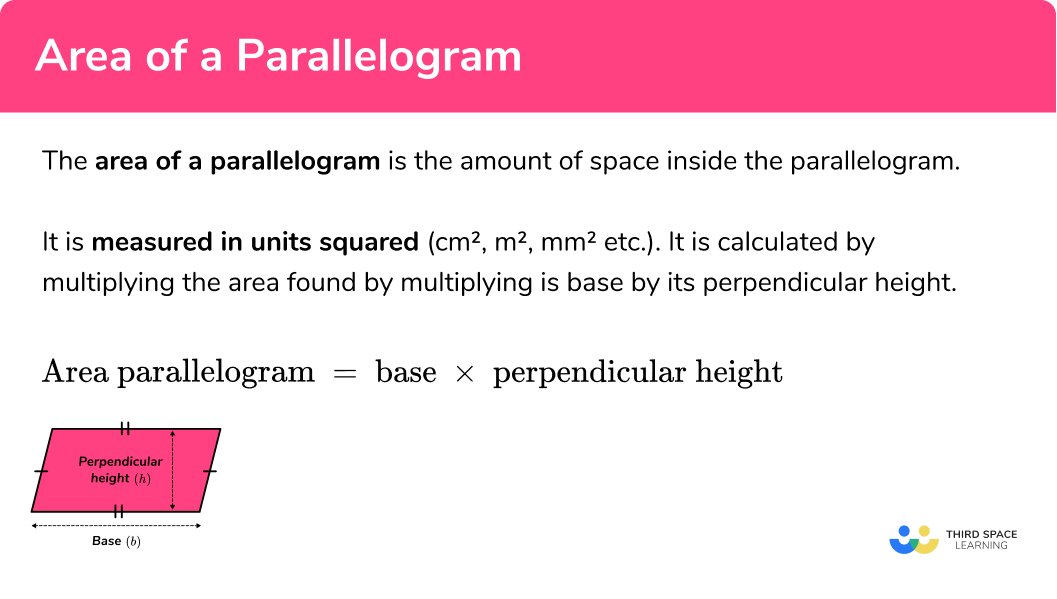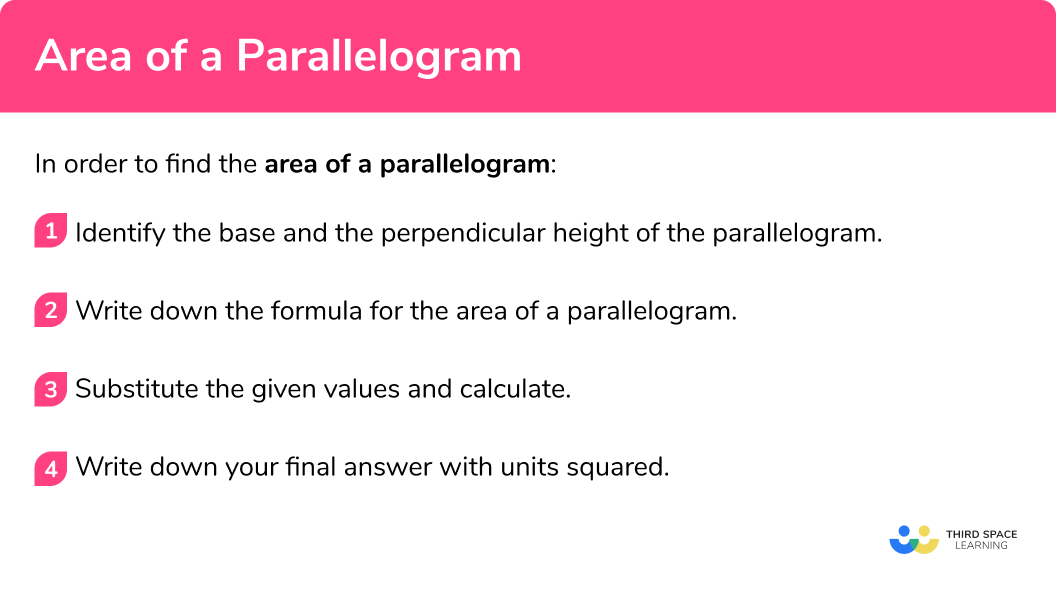GCSE Maths Geometry and Measure Area

Area Of A Parallelogram

# Area Of A Parallelogram

Here we will learn how to find the area of a parallelogram, including compound area questions, questions with missing side lengths and questions involving unit conversion.

There are also area of a area of a parallelogram worksheets based on Edexcel, AQA and OCR exam questions, along with further guidance on where to go next if you’re still stuck.

## What is the area of a parallelogram?

The area of a parallelogram is the amount of space inside a parallelogram. It is measured in units squared ( cm^{2}, m^{2}, mm^{2} etc.)

A parallelogram is a quadrilateral ( 4 sided shape) with 2 pairs of parallel sides. The parallel sides are the same length and the opposite angles are equal. The interior angles of the parallelogram add up to 360° .

The area of a parallelogram is calculated by multiplying the base of a parallelogram by its perpendicular height.

Area of a parallelogram formula:

\begin{aligned} \text { Area }_{\text {parallelogram }}&=\text { base } \times \text { perpendicular height } \\ \text { Area }_{\text {parallelogram }}&=b \times h \end{aligned}

Let’s see why this works:

If we cut off the triangle at one end of a parallelogram and attach it to the other we form a rectangle. The formula for the area of a rectangle is length\times width (or in this case base \times height).

Our final answer must be in units squared
E.g. square centimetres ( cm^{2}) , square metres ( m^{2} ), square feet ( ft^{2} ) etc.

### What is the area of a parallelogram?## Parallelogram

A parallelogram is a 2D shape with opposite sides that are both parallel and equal in length. The diagonals of a parallelogram bisect each other. We can categorise them as quadrilaterals because they have 4 sides.

We need to be able to identify the three special types of parallelograms.

E.g.

A parallelogram where all sides are equal is a rhombus.

E.g.

A parallelogram in which all angles are right angles, and the diagonals are equal is a rectangle.

E.g.

A parallelogram with all equal sides and all angles equal to 90 degree, and equal diagonals is a square.

A parallelogram is, essentially, a slanted rectangle, so we can use the same calculation to find the area of a parallelogram as you would calculate the area of a rectangle, as long as you use the perpendicular height rather than the slant length.

E.g.

Calculate the area of the parallelogram.

Area = 10 x 6 = 60

Let’s explore more about parallelograms below.

## How to find the area of a parallelogram

In order to find the area of a parallelogram:

1. Identify the base and the perpendicular height of the parallelogram.
2. Write down the formula for the area of a parallelogram.
3. Substitute the given values and calculate.

### How to find the area of a parallelogram## Related lessons on area

Area of a parallelogram is part of our series of lessons to support revision on area. You may find it helpful to start with the main area lesson for a summary of what to expect, or use the step by step guides below for further detail on individual topics. Other lessons in this series include:

## Area of a parallelogram examples

### Example 1: finding area given the base and perpendicular height

Find the area of the parallelogram below:

1. Identify the base and perpendicular height of the parallelogram.

Base = 10m

Perpendicular height = 6m

2Write down the formula for the area of a parallelogram.

\text { Area }_{ \text { parallelogram }}=\text { Base } \times \text { Perpendicular Height }

3Substitute the given values and calculate.

\begin{aligned} \text { Area }_{\text {parallelogram }}&=\text { Base } \times \text { Perpendicular Height } \\ &=10 \times 6 \\ &=60 \end{aligned}

\text { Area }_{\text {parallelogram }}=60 \mathrm{~m}^{2}

### Example 2: finding the area of a parallelogram requiring converting units

Find the area of the parallelogram below:

Identify the base and perpendicular height of the parallelogram.

Write down the formula for the area of a parallelogram.

Substitute the given values and calculate.

### Example 3: worded question

A painter is painting a logo on the side of an office block. The logo is composed of four identical parallelograms and is shown below. Each tin of paint costs £1.20 and contains enough paint to cover an area of 2.5m^{2} . How much will it cost to paint the logo?

Identify the base and perpendicular height of the parallelogram.

Write down the formula for the area of a parallelogram.

Substitute the given values and calculate.

### Example 4: calculating base length given the area

Find the base length of the parallelogram below:

Identify the base and perpendicular height of the parallelogram.

Write down the formula for the area of a parallelogram.

Substitute the given values and calculate.

### Example 5: compound area

Calculate the area of the shaded region below:

Identify the base and perpendicular height of the parallelogram.

Write down the formula for the area of a parallelogram.

Substitute the given values and calculate.

### Example 6: finding the area involving algebra question

Calculate the value of x in the parallelogram below:

Identify the base and perpendicular height of the parallelogram.

Write down the formula for the area of a parallelogram.

Substitute the given values and calculate.

### Common misconceptions

• Using incorrect units for the answer

A common error is to forget to include squared units when asked to calculate area.

• Forgetting to convert measures to a common unit

Before using the formula for calculating the area of a parallelogram, we need to ensure the units used are the same. If different units are given (E.g. length = 4m and width = 3cm ) we must convert them both to either cm or both to m .

• Length of the slanted side and perpendicular height

Sometimes in a question we are given additional lengths which are not needed in our calculations. An example of this is being given the length of one of the slanted sides of the parallelogram. To calculate the area we must ignore the slant length and instead use the vertical height.

### Practice Area of a parallelogram questions

1. Find the area of the parallelogram below:17.5m66m^{2}17.5m66mb \times  h = 12 \times 5.5 = 66m^{2}. Remember to have units squared in your final answer.

2. Find the area of the parallelogram below:31.5m^{2}3150m^{2}22.4m^{2}14.4m^{2}Convert 450cm into metres by dividing by 100 . Multiply the base length (7m) by the converted perpendicular height (4.5m) to equal 31.5m^{2} . Be careful not to multiply by the length of the diagonal instead of the perpendicular height.

3. Calculate the area of the garden below:25.2m^{2}12.2m^{2}31.2m^{2}37.2m^{2}Find the area of the parallelogram by multiplying the base length and the height. Find the area of the triangle by multiplying the base and the height and dividing by 2 . Add together the area of the triangle and parallelogram.

4. Calculate the length of the base of the parallelogram below:22cm2662cm^{2}22cm^{2}2662cmDivide the area by the perpendicular height of 11m . Remember that you are not calculating the area so your final answer should not be in units squared.

5. Calculate the area of the shaded region below:126cm^{2}224cm^{2}98cm^{2}126.98cm^{2}Convert all measures to cm . Find the area of the parallelogram first by multiplying the base times the height. Add on the area of the rectangle which is calculated by multiplying the length and the width.

6. Calculate the base length in the parallelogram below:4cm5cm16cm33cmMultiply the base times the perpendicular height, Remember to multiply the two by both terms. Rearrange the equation to calculate x . Substitute the value of x back into 4x-11 to calculate the base length.

### Area of a parallelogram GCSE questions

1. Find the area of the parallelogram. Remember to show your full working.(2 marks)

38\times19

(1)

722m^{2}

(1)

2.  A landscaper is planning a rectangular garden for a client. The garden will feature a patio and sandpit both in the shape of parallelograms. The remainder of the garden will be covered in grass. Below is a blueprint of the garden.
(a)What area of the garden will be covered in grass?(b) A roll of grass covers an area of 3m^{2} and costs £14.50 . How much will it cost to put grass in the garden?

(7 marks)

(a)
Area of rectangle = 15\times7 = 105

(1)

Area of both parallelograms: 5\times4 = 20 and 4.5\times1.5 = 6.75

(1)

Area of rectangle – area of parallelograms=  105 – 20 – 6.75

(1)

78.25m^{2}

(1)

(b)
78.25\div3 = 26.08 rolls of grass

(1)

27\times14.5

(1)

£391.50

(1)

3. Calculate the value of x in the diagram below:(4 marks)

6 \times (3x-9)

(1)

18x-54=36

(1)

Evidence of rearranging equation to solve for x

(1)

x = 5

(1)

## Learning checklist

You have now learned how to:

• Calculate and compare the area of parallelograms using standard units
• Calculate the area of parallelograms and related composite shapes

## Still stuck?

Prepare your KS4 students for maths GCSEs success with Third Space Learning. Weekly online one to one GCSE maths revision lessons delivered by expert maths tutors.

Find out more about our GCSE maths tuition programme.